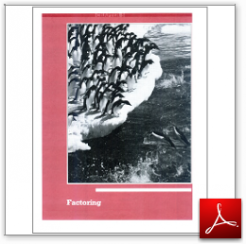Algebra Math Book - Introductory Algebra Thank you for your continual support.

 The power of mathematics rests in the logic of thinking.ID: Chapter05
Price: 9.95

# Algebra Math book - Introductory Algebra - Chapter 5 - Factoring

## Chapter 5 of this algebra math book has 8 sections.

To get started, you might like to take a free test just to see what areas you might need to study.  Take the Test.

 Sec5.1 Section5.1-Factoring Polynomials Sec5.2 Section5.2-Difference of Squares and Perfect Square Trinomials Sec5.3 Section5.3-Factoring Trinomials of the Type Sec5.4 Section5.4-Factoring Trinomials of the Type Sec5.5 Section5.5-A Strategy for Factoring Completely Sec5.6 Section5.6-Solving Equations by Factoring Sec5.7 Section5.7-Applications Sec5.8 Section5.8-Factoring the Sum and Difference of Two Cubes

For a complete listing of the objectives in this chapter visit the List of Objectives.

This section of my algebra math book, Introductory Algebra, also includes in the download:

• Cover Sheet